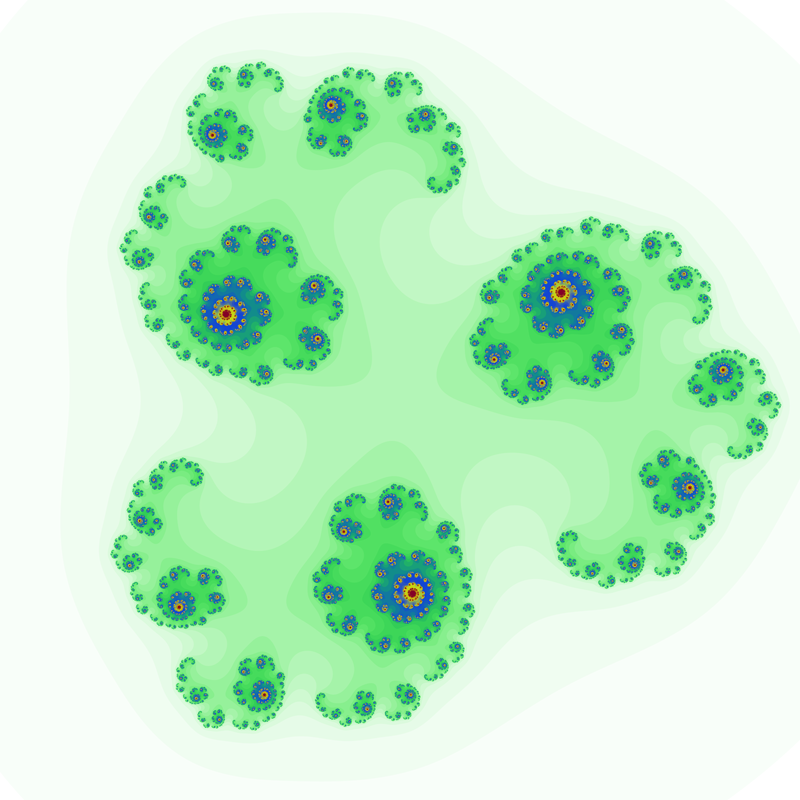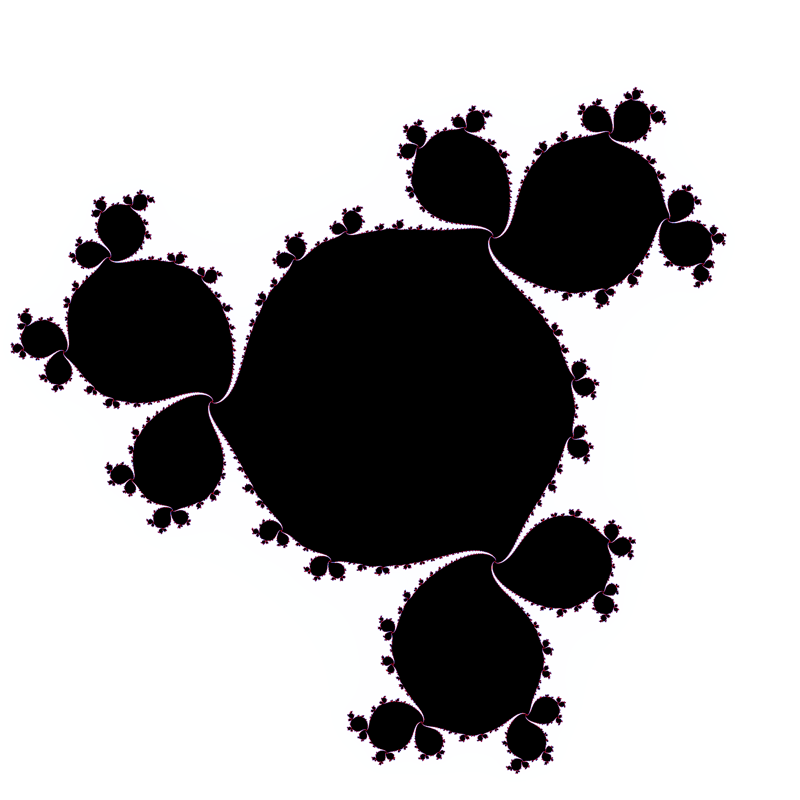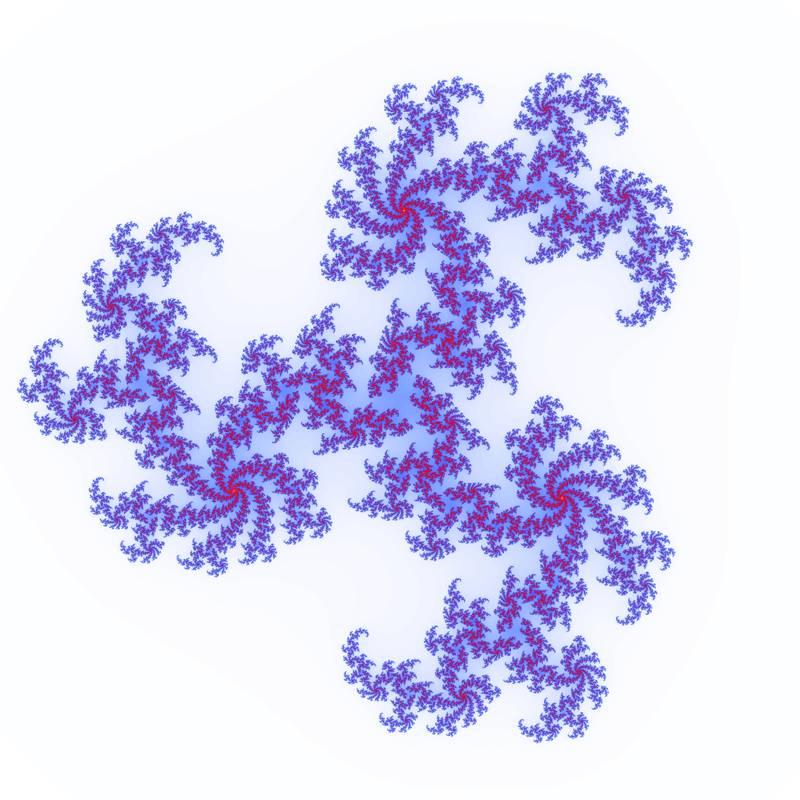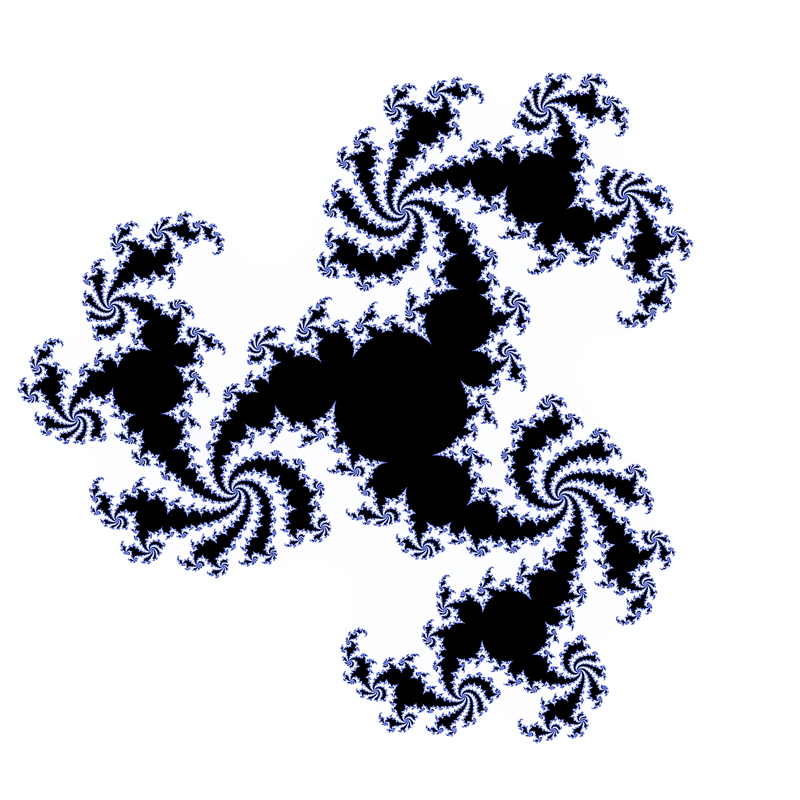# Cubic Julia set

Written by Paul Bourke
March 2002

Illustration of the behavour of the series for points z0 on the complex plane.Each value of "c" gives a different fractal image, common value is -(0.5 + 0.05 i).c = 0.5 + 0.5 ic = 0.7 + 0.5 ic = 0.53 + 0.1 ic = 0.52 + 0.1 ic = 0.515 + 0.1 i